Search by Topic

Resources tagged with Maths Supporting SET similar to Poiseuille's Equation:

Filter by: Content type:
Age range:
Challenge level:

There are 89 results

Broad Topics > Applications > Maths Supporting SETApproximately Certain

Age 14 to 18 Challenge Level:

Estimate these curious quantities sufficiently accurately that you can rank them in order of sizePopulation Dynamics Collection

Age 16 to 18 Challenge Level:

This is our collection of tasks on the mathematical theme of 'Population Dynamics' for advanced students and those interested in mathematical modelling.Big and Small Numbers in the Physical World

Age 14 to 16 Challenge Level:

Work with numbers big and small to estimate and calculate various quantities in physical contexts.Guessing the Graph

Age 14 to 16 Challenge Level:

Can you suggest a curve to fit some experimental data? Can you work out where the data might have come from?Global Warming

Age 14 to 16 Challenge Level:

How much energy has gone into warming the planet?Bessel's Equation

Age 16 to 18 Challenge Level:

Get further into power series using the fascinating Bessel's equation.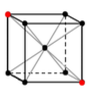Crystal Symmetry

Age 16 to 18 Challenge Level:

Use vectors and matrices to explore the symmetries of crystals.Real-life Equations

Age 16 to 18 Challenge Level:

Here are several equations from real life. Can you work out which measurements are possible from each equation?A Question of Scale

Age 14 to 16 Challenge Level:

Use your skill and knowledge to place various scientific lengths in order of size. Can you judge the length of objects with sizes ranging from 1 Angstrom to 1 million km with no wrong attempts?Constantly Changing

Age 14 to 16 Challenge Level:

Many physical constants are only known to a certain accuracy. Explore the numerical error bounds in the mass of water and its constituents.Stirling Work

Age 16 to 18 Challenge Level:

See how enormously large quantities can cancel out to give a good approximation to the factorial function.Pdf Stories

Age 16 to 18 Challenge Level:

Invent scenarios which would give rise to these probability density functions.Big and Small Numbers in the Living World

Age 11 to 16 Challenge Level:

Work with numbers big and small to estimate and calculate various quantities in biological contexts.The Wrong Stats

Age 16 to 18 Challenge Level:

Why MUST these statistical statements probably be at least a little bit wrong?Whose Line Graph Is it Anyway?

Age 16 to 18 Challenge Level:

Which line graph, equations and physical processes go together?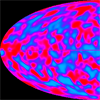Big and Small Numbers in Physics

Age 14 to 16 Challenge Level:

Work out the numerical values for these physical quantities.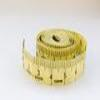Bigger or Smaller?

Age 14 to 16 Challenge Level:

When you change the units, do the numbers get bigger or smaller?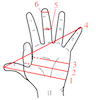Age 14 to 16 Challenge Level:

Which units would you choose best to fit these situations?Big and Small Numbers in Chemistry

Age 14 to 16 Challenge Level:

Get some practice using big and small numbers in chemistry.Far Horizon

Age 14 to 16 Challenge Level:

An observer is on top of a lighthouse. How far from the foot of the lighthouse is the horizon that the observer can see?Over-booking

Age 16 to 18 Challenge Level:

The probability that a passenger books a flight and does not turn up is 0.05. For an aeroplane with 400 seats how many tickets can be sold so that only 1% of flights are over-booked?Scale Invariance

Age 16 to 18 Challenge Level:

By exploring the concept of scale invariance, find the probability that a random piece of real data begins with a 1.Building Approximations for Sin(x)

Age 16 to 18 Challenge Level:

Build up the concept of the Taylor seriesDesigning Table Mats

Age 11 to 16 Challenge Level:

Formulate and investigate a simple mathematical model for the design of a table mat.Dangerous Driver?

Age 16 to 18 Challenge Level:

Was it possible that this dangerous driving penalty was issued in error?Debt Race

Age 16 to 18 Challenge Level:

Who will be the first investor to pay off their debt?Perfect Eclipse

Age 14 to 16 Challenge Level:

Use trigonometry to determine whether solar eclipses on earth can be perfect.Investigating the Dilution Series

Age 14 to 16 Challenge Level:

Which dilutions can you make using only 10ml pipettes?Reaction Rates

Age 16 to 18 Challenge Level:

Explore the possibilities for reaction rates versus concentrations with this non-linear differential equationConstruct the Solar System

Age 14 to 18 Challenge Level:

Make an accurate diagram of the solar system and explore the concept of a grand conjunction.Carbon Footprints

Age 14 to 16 Challenge Level:

Is it really greener to go on the bus, or to buy local?Stats Statements

Age 16 to 18 Challenge Level:

Are these statistical statements sometimes, always or never true? Or it is impossible to say?Taking Trigonometry Series-ly

Age 16 to 18 Challenge Level:

Look at the advanced way of viewing sin and cos through their power series.Vector Walk

Age 14 to 18 Challenge Level:

Starting with two basic vector steps, which destinations can you reach on a vector walk?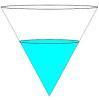Fill Me up Too

Age 14 to 16 Challenge Level:

In Fill Me Up we invited you to sketch graphs as vessels are filled with water. Can you work out the equations of the graphs?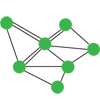Investigating Epidemics

Age 11 to 16 Challenge Level:

Simple models which help us to investigate how epidemics grow and die out.Truth Tables and Electronic Circuits

Age 11 to 18

Investigate circuits and record your findings in this simple introduction to truth tables and logic.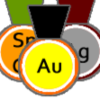Alternative Record Book

Age 14 to 18 Challenge Level:

In which Olympic event does a human travel fastest? Decide which events to include in your Alternative Record Book.Polygon Walk

Age 16 to 18 Challenge Level:

Go on a vector walk and determine which points on the walk are closest to the origin.Fix Me or Crush Me

Age 16 to 18 Challenge Level:

Can you make matrices which will fix one lucky vector and crush another to zero?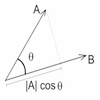Cross with the Scalar Product

Age 16 to 18 Challenge Level:

Explore the meaning of the scalar and vector cross products and see how the two are related.Scientific Curves

Age 16 to 18 Challenge Level:

Can you sketch these difficult curves, which have uses in mathematical modelling?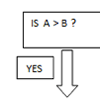Mystery Procedure

Age 14 to 16 Challenge Level:

Can you work out what this procedure is doing?Transformations for 10

Age 16 to 18 Challenge Level:

Explore the properties of matrix transformations with these 10 stimulating questions.Square Pair

Age 16 to 18 Challenge Level:

Explore the shape of a square after it is transformed by the action of a matrix.Matrix Meaning

Age 16 to 18 Challenge Level:

Explore the meaning behind the algebra and geometry of matrices with these 10 individual problems.Nine Eigen

Age 16 to 18 Challenge Level:

Explore how matrices can fix vectors and vector directions.How Do You React?

Age 14 to 16 Challenge Level:

To investigate the relationship between the distance the ruler drops and the time taken, we need to do some mathematical modelling...Robot Camera

Age 14 to 16 Challenge Level:

Could nanotechnology be used to see if an artery is blocked? Or is this just science fiction?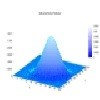Pdf Matcher

Age 16 to 18 Challenge Level:

Which pdfs match the curves?